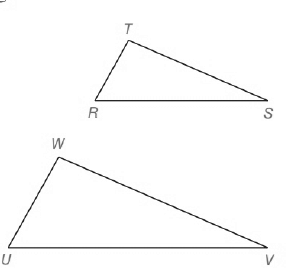Chapter 5.CT, Problem 4CTElementary Geometry For College St...

7th Edition
Alexander + 2 others
ISBN: 9781337614085

Solutions

Chapter
SectionElementary Geometry For College St...

7th Edition
Alexander + 2 others
ISBN: 9781337614085
Textbook Problem

△ R T S ~ △ U W V .a) Find m ∠ W if m ∠ R = 67 ° and m ∠ S = 21 ° .b) Find WV if R T = 4 ,   U W = 6 ,   a n d   T S = 8 .To determine

a)

To find:

The measure of angle W.

Explanation

Given, RTS~UWV i.e both triangles are similar to each other.

mR=67° and mS=21°.

Sum of the angles of triangle =180°

Consider, RTS

R+T+S=180°

To determine

b)

To find:

The length of WV.

Still sussing out bartleby?

Check out a sample textbook solution.

See a sample solution

The Solution to Your Study Problems

Bartleby provides explanations to thousands of textbook problems written by our experts, many with advanced degrees!

Get Started

In Exercises 1-6, simplify the expression. 818

Calculus: An Applied Approach (MindTap Course List)

Fill in each blank: 88oz=lb

Elementary Technical Mathematics

limx5x225x29x+20= a) 1 b) 10 c) d) does not exist

Study Guide for Stewart's Single Variable Calculus: Early Transcendentals, 8th

The length of a = 4i – j – 2k is: 11 21

Study Guide for Stewart's Multivariable Calculus, 8th